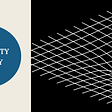# What are the gas laws and their formulas?

An ideal gas is a theoretical gas that does not exist in reality but is assumed to exist for the purpose of simplifying calculations. An ideal gas is a gas in which particles are moving randomly as all gases do, the only difference being that for an ideal gas when its particles collide with each other, these collisions are considered as perfectly elastic collision which means no energy of either of these particles is loses it differs ideal gases from the real gases. Real gases can be derived by van der Waals equation.

In reality, however, when actual gas particles collide with each other, some of their kinetic energy is lost in changing directions and overcoming friction. However, at NTP conditions most natural gases like methane, ethane, propane, etc act just like an ideal gas. All the gas behaves similarly to an ideal gas under the conditions of high temperature and low pressure. To derive the ideal gas law first we know the properties of gases and gas laws to the formulation of the ideal gas equation.

## What is the mathematical expression for Boyle’s Law?

The absolute pressure exerted by a given mass of an ideal gas is inversely proportional to the volume it occupies if both the temperature and amount of gas remain unchanged. Mathematically this law is given as P ∝ 1/V, or PV = K, where P = pressure of the gas; V= volume of the gas; K= gas constant.

## What is the formula for the Charles Law?

When the pressure of a sample of air is held constant, then the volume of the gas is directly proportional to its temperature.
Mathematically this law is given as, V ∝ T, or, V = KT, where V= Volume of a gas sample; T= Absolute temperature.

Equal volumes of all gases at conditions of the same temperature and pressure have the same number of molecules. Mathematically this law is given as, V ∝ n, or, V/n =K, where V=volume of gas; n= number of moles.

## Ideal gas equation derivation

If we combine the results of all the above gas laws we get an equation that holds true for an ideal gas. The most common form of this equation is since PV= K and V/T =k, then PV/T = constant. Thus, the ideal gas equation is,
PV = nRT.

--

--

--

## More from Learning Chemistry Online

This publication of physical chemistry is restricted so as to include the application of principles in the form of solved problems and numerical.

## How To Learn Algebra## The Chain Rule of Conditional Probabilities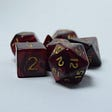## The story of time complexity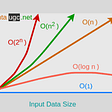## Binomial Coefficients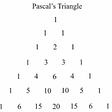## Why gigantic numbers baffle the mind## The MFT System Part 3: Detection## The Monty Hall Problem — Understanding Through Exaggeration## Chemistry Topics

Chemistry is the study of properties, structure, composition of atoms, ions, and molecules. This materials provides different Chemistry topics and questions

## IMMERMAN–SZELEPCSÉNYI THEOREM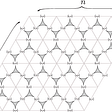## Interview with an 🖤 Empath## Why do we need Quantum Gravity?